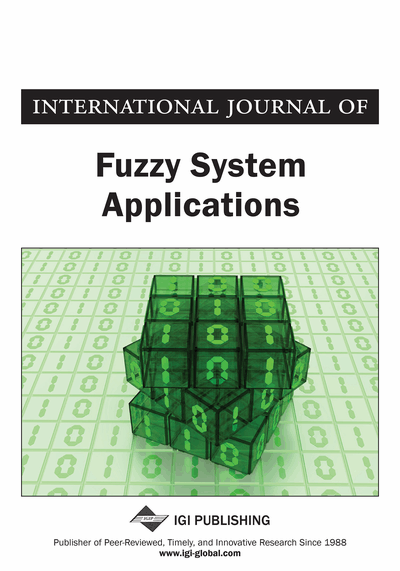# Bector-Chandra Type Duality in Linear Programming Under Fuzzy Environment Using Hyperbolic Tangent Membership Functions

Pratiksha Saxena, Ravi Jain
DOI: 10.4018/IJFSA.2019040104
OnDemand:
(Individual Articles)
Available
\$37.50
No Current Special Offers

## Abstract

Multi-objective optimization has been applied in many fields of science, including engineering, economics and logistics where optimal decisions need to be taken in the presence of trade-offs between two or more conflicting objectives. One approach to optimize a multi-objective mathematical model is to employ utility functions for the objectives. Recent studies on utility-based multi-objective optimization concentrates on considering just one utility function for each objective. But, in reality, it is not reasonable to have a unique utility function corresponding to each objective function. Here, a constrained multi-objective mathematical model is considered in which several utility functions are associated for each objective. All of these utility functions are uncertain and in fuzzy form, so a fuzzy probabilistic approach is incorporated to investigate the uncertainty of the utility functions for each objective. Meanwhile, the total utility function of the problem will be a fuzzy nonlinear mathematical model. Since there are not any conventional approaches to solve such a model, a defuzzification method to change the total utility function to a crisp nonlinear model is employed. Also, a maximum technique is applied to defuzzify the conditional utility functions. This action results in changing the total utility function to a crisp single objective nonlinear model and will simplify the optimization process of the total utility function. The effectiveness of the proposed approach is shown by solving a test problem.
Article Preview
Top

## 1. Introduction

Linear programming is one of the most frequently applied operations research models for solving real world problems. A large number of problems in production, planning and scheduling, transportation, location, finance and engineering design may be cast into a linear programming framework. The linear programming models are used for solving real world decision making problems with the objective of maximization/minimization of a linear objective function under linear constraints. The linear programming technique is based on the assumptions of proportionality, additivity and certainty. But in many real-life situations, the decision maker may not be able to specify the objective and/or constraint functions precisely but rather can specify them in fuzzy sense. The fuzziness may appear in linear programming model in many ways, (i) the inequalities may be fuzzy and/or goal may be fuzzy (ii) the model parameters may be in terms of fuzzy numbers. For (i) kind of fuzzy linear programming problems, the limit of fuzziness is set by the decision maker in terms of his/her aspiration levels and for (ii) kind of fuzzy linear programming problems, the feasible and optimal solutions are defined on the basis of fuzzy relations.

The concept of fuzzy sets is introduced by Zadeh (1965) in 1965, the researchers have advanced it in a variety of ways and in many disciplines. Its applications can be found, for example, in computer science, decision theory, expert systems, operations research, pattern recognition, artificial intelligence and optimization. Decision making in fuzzy environment was first proposed by Bellman and Zadeh (1970). Subsequently, Tanaka et al. (1984) made use of their concept of decision making in fuzzy environment for mathematical programming. Since then the fuzzy set theory has been extensively used to capture linguistic uncertainty in optimization problems. A number of researchers have shown their interest to handle this kind of uncertainty in the class of fuzzy mathematical programming, (e.g., see Hamacher et al., 1978), (Zimmermann, 1978), (Zimmermann, 2001), (Gupta & Mehlawat, 2009), (Saxena & Jain, 2014) and (Lai & Hwang, 1992).

However, a vast literature is available on modelling and solution procedures for a fuzzy linear programming problem but only a few researchers exhibit their interest in duality in fuzzy linear programming. The most basic results available on duality in linear programming under fuzzy environment are due to Hamacher et al. (1978) and Rödder and Zimmermann (1980). In recent years, several authors have worked on duality in (i) linear programming (Gupta & Mehlawat, 2009), (Saxena & Jain, 2014), (Bector & Chandra, 2002) (ii) quadratic programming (Bector & Chandra, 2005) (iii) convex fractional programming (Gupta & Mehlawat, 2009), (Saxena & Jain, 2017) under fuzzy environment and established duality results.

## Complete Article List

Search this Journal:
Reset
Volume 13: 1 Issue (2024)
Volume 12: 1 Issue (2023)
Volume 11: 4 Issues (2022)
Volume 10: 4 Issues (2021)
Volume 9: 4 Issues (2020)
Volume 8: 4 Issues (2019)
Volume 7: 4 Issues (2018)
Volume 6: 4 Issues (2017)
Volume 5: 4 Issues (2016)
Volume 4: 4 Issues (2015)
Volume 3: 4 Issues (2013)
Volume 2: 4 Issues (2012)
Volume 1: 4 Issues (2011)
View Complete Journal Contents Listing